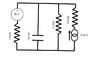# RC circuit with dc current source

• Engineering
• Melizard0
In summary, the problem asks to solve for the current in each branch of the circuit as a function of time, using the mesh method. The second part asks for the voltage across the current source as a function of time. To solve this, initial conditions may be needed and it may be treated as an AC circuit with a function for current instead of a constant value.

## Homework Statement

Solve for the current in each branch of the following circuit as a function of time & derive and expression for the time dependence of teh emf of the constant current source in the circuit below.

## Homework Equations

Zc = Xc = 1/(wC) = t/(2pi*C)

## The Attempt at a Solution

I attempted to use the mesh method:

Loop 1:
-1000(i1)+50-(Zc)(i1)+(Zc)(i2)=0

Loop 2
-(Zc)(i2)+(Zc)(i1)-3500(i2)+3500(i3)=0

Loop3:
-3500(i3)+3500(i2)-5000(i3)-V=0

This quickly got confusing when I substituted Zc for t/(2pi*120mF).
I have no idea how to solve this problem when it has both a capacitor and a current source.

I also do not understand what the second part of the question is asking.

I would appreciate any help/insight that you could offer. Thanks.

#### Attachments

•electronics.jpg
19.6 KB · Views: 580
We can't see your picture until it's been approved, but I can tell you that for these kind of questions, usually, you have to either use differential equations or Laplace transformations. I assume you're familiar with one of them?

The second part gets me too. I can understand each word separately, but put them together and it's jibberish to me.The 2nd part asks for the voltage across the current source, as a function of time.

As a start, you could get the steady-state currents and voltages (t→∞).

To solve this, I think we need some initial condition that isn't given. For example, perhaps the capacitor voltage is zero at t=0?

That's all the information that I'm given. I have a feeling that since the current varies with time that I have to treat it like an ac circuit, and put in a function instead of i. I'm meeting with my prof today, so hopefully he provides some more insight.

## 1. What is an RC circuit with a DC current source?

An RC circuit with a DC current source is a circuit that contains a resistor (R) and a capacitor (C) connected in series with a direct current (DC) source. The capacitor acts as a temporary storage for electrical charge, while the resistor limits the flow of current in the circuit.

## 2. How does an RC circuit with a DC current source work?

In an RC circuit with a DC current source, the capacitor charges up to the same voltage as the source, and then discharges through the resistor. This process is repeated continuously, creating a current flow in the circuit. The rate of charging and discharging depends on the values of the resistor and capacitor.

## 3. What is the time constant of an RC circuit with a DC current source?

The time constant (τ) is a measure of how quickly the capacitor charges and discharges in an RC circuit with a DC current source. It is calculated by multiplying the resistance (R) in ohms by the capacitance (C) in farads (τ = RC). The time constant determines the speed at which the circuit reaches a steady-state.

## 4. How does changing the values of R and C affect an RC circuit with a DC current source?

Changing the values of R and C in an RC circuit with a DC current source can have different effects on the circuit. Increasing the resistance will slow down the rate of charging and discharging, while decreasing the resistance will speed it up. Similarly, increasing the capacitance will increase the time constant and decrease the frequency of charging and discharging, while decreasing the capacitance will have the opposite effect.

## 5. What are some practical applications of RC circuits with DC current sources?

RC circuits with DC current sources have many practical applications, such as in electronic filters, time-delay circuits, and power supplies. They are also commonly used in electronic devices to smooth out voltage fluctuations caused by the AC current from the power source. Additionally, they can be used in signal processing and audio amplifiers to remove unwanted frequencies.